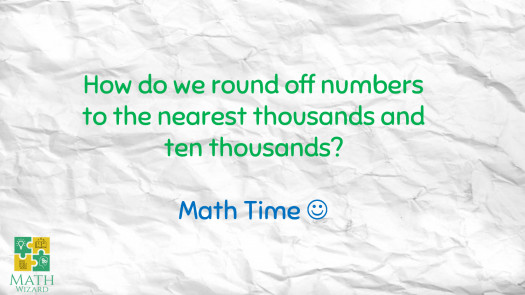# Rounding Off Numbers (Assimilation)

5 Questions | Total Attempts: 1306Settings• 1.
In rounding off 56 327 to the nearest thousands, are you going to round up or round down?
• A.

Round up

• B.

Round down

• C.

None of the above

• 2.
In rounding off 56 327 to the nearest ten thousands, are you going to round up or round down?
• A.

Round up

• B.

Round down

• C.

None of the above

• 3.
In rounding off 87 099 to the nearest thousands, the answer is 88 000.
• A.

True

• B.

False

• 4.
In rounding off 50 999 to the nearest ten thousands, the answer is 50 000.
• A.

True

• B.

False

• 5.
How do we round off numbers?
• A.

Underline the digit being rounded, look to the right. If its 5 to 9 round down. If its 4 to 0 round up.

• B.

Underline the digit being rounded, look to the right. If its 5 to 9 round up (add 1). If its 4 to 0 round down.

• C.

Underline the digit being rounded. If its 5 to 9 round up (add 1). If its 4 to 0 round down.

• D.

Underline the digit being rounded. If its 5 to 9 round down. If its 4 to 0 round up.

Related TopicsBack to top